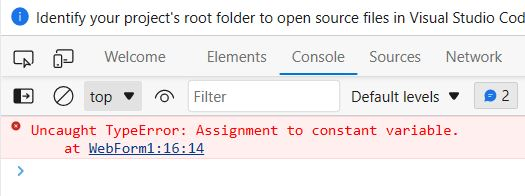﻿ JavaScript Variables - TutorialsTrend
This Angular tutorial helps you get started with Angular quickly and effectively through many practical examples.
`

# JavaScript Variables

Variables are the fundamental of all programming languages. Variables are used to store data such as strings of text, integers, and so on. The variables' data or values can be set, modified, and retrieved as needed.

In JavaScript, var keyword is used to declare variables since JavaScript was created. After that ES6 introduces two new keywords let and const for declaring variables.

#### JavaScript Variable Nameing Conventions

1. Variable names are case-sensitive in JavaScript. So, the variable names msg, Myvar, myVar, MyVar are considered separate variables.
2. Variable names can contain letters, digits, or the symbols \$ and _.
4. A variable name cannot be a reserved keyword in JavaScript, e.g. var, function, return cannot be variable names.

#### 1. JavaScript Var Variable

The var keyword declares a function-scoped or global variable, optionally initializing it to a value. Function-scoped means that the variable is only available within the function it was declared in. Global variables are available throughout your entire code.

Using Var keyword variable can be define in two scope Global and Function Scope as following:

• Function- variable declare with in the function. So it is the local to that function is called local or function scope.
• Global- variable declared outside the function. So variable becomes global.

You can create a variable with the var keyword, whereas the assignment operator (=) is used to assign value to a variable, like this: var varName = value;

Example

``````<!DOCTYPE html>
<html lang="en">
<meta charset="utf-8">
<body>
<script>
// Creating variables
var name = "Rahul";
var age = 26;
var email = "rahul@gmail.com";

// Printing variable values
document.write(name + "<br>");
document.write(age + "<br>");
document.write(email);
</script>
</body>
</html>``````

#### 2. JavaScript let Variable

The let keyword declares a block-scoped local variable, optionally initializing it to a value. Block-scoped means that the variable is only available within the block it was declared in, which is usually denoted by curly braces {}.

Example

You can create a let with the let keyword, whereas the assignment operator (=) is used to assign value to a variable, like this: let varName = value;

``````<!DOCTYPE html>
<html lang="en">
<meta charset="utf-8">
<body>
<p><b>let variable example</b></p>
<script>
// Creating variables
let name = "Rahul";
let age = 26;
let email = "rahul@gmail.com";

// Printing variable values
document.write(name + "<br>");
document.write(age + "<br>");
document.write(email);
</script>
</body>
</html>``````

#### 3. JavaScript const Variable

The const keyword declares a block-scoped, immutable constant variable, i.e. a variable that can’t be reassigned. Constants are also called immutable variables, but that’s a bit of a misnomer since they are actually variables – just ones that can’t be reassigned.

Example

``````<!DOCTYPE html>
<html lang="en">
<meta charset="utf-8">
<body>
<p><b>const variable example</b></p>
<script>
// Creating variables
const name = "Rahul";
const age = 26;
const email = "rahul@gmail.com";

name = "mahesh"; // reassign the const variable

// Printing variable values
document.write(name + "<br>");
document.write(age + "<br>");
document.write(email);
</script>
</body>
</html>``````

If you look at the browser console by clicking the F12 key on your keyboard, you'll notice something like that:

OutputPrev Next